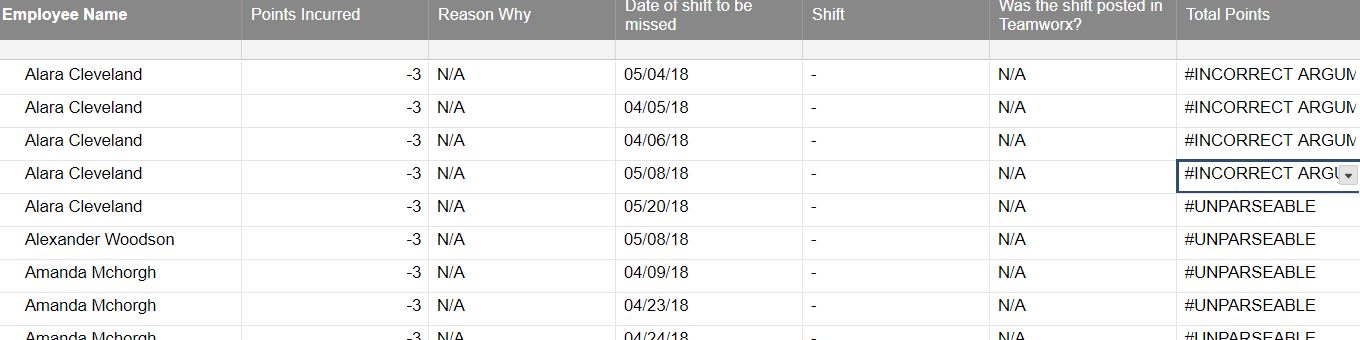# How to total quantity based on similar name in column

I am trying to total points based on the persons name.  Basically one column has the names, some of them duplicates.  Another Column has points.  I need a third column for total points at the end that looks at the names, finds those that are the same and totals the points for that person in the total points column.  I have done this in excel

=SUMPRODUCT((\$B\$2:\$B\$4900=B2)*(\$C\$2:\$C\$4900))

Column B is the name

Column C is the points

This formula is in the total points column.

I tried doing this in excel and then uploading to smartsheets but I get the #unparseable error.  Any help would be greatly appreciated.• try

=sum(Collect(

keep in mind collect is a little weird. It gets an array from one column based on criteria in other columns. The first reference is the return column, then you reference a range for criteria, and the criteria. Once you've built your array with collect, sum will add all of the values together.

• you could also try a sumif formula

in row 1 =SUMIF([ColumnB]:[ColumnB], [ColumnB]1, [ColumnC]:[ColumnC])

the formula should pick up the name in ColumnB for each specific row and use it as the criteria

## Help Article Resources

Want to practice working with formulas directly in Smartsheet?

Check out the Formula Handbook template!